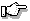# PgDrawSpan(), PgDrawSpanmx()

Draw a list of spans

## Synopsis:

```int PgDrawSpan( PgSpan_t const *ptr,
int num,
PhPoint_t const *pos,
int flags );

int PgDrawSpanmx( PgSpan_t const *ptr,
int num,
PhPoint_t const *pos,
int flags );```

## Description:

These low-level draw primitives let you render complex shapes not supported by the Photon graphics drivers.

The functions draw a list of spans. The spans are defined as a list of PgSpan_t records. Here are the members of PgSpan_t:

short x1
starting x position
short x2
last x position
short y
y position

The number of spans is defined by the num parameter. The location of the spans is offset by the pos parameter.

You can set flags to one of the following:

• Pg_DRAW_FILL - draw with fill parameters.
• Pg_DRAW_STROKE - draw with stroke parameters.
• Pg_DRAW_TEXT - draw with text parameters.If you call the "mx" form of this function, the data isn't physically copied into the draw buffer. Instead, a pointer to the list of spans is stored until the draw buffer is flushed. Make sure you call PgFlush() before you modify the list.

## Returns:

0
Success.
-1
The draw buffer is too small to hold the current draw state, the draw command and the data. Increase the size of the draw buffer, or decrease the number of points.

## Examples:

The following example:

```void DrawSpan() {
PgSpan_t    spans;
PgSpan_t    *sp = spans;
PhPoint_t   p = { 12, 10 };
int         i, v, n=0;

for (i=0; i<=100; i++) {
sp->x1 = (i*i)>>6;
v = 100 - i;
sp->x2 = 160 - ((v*v)>>6);
sp->y = i;
sp++; n++;
}
for (i=0; i<=50; i++) {
sp->x1 = 100 - ((i*i)>>6);
v = 50 - i;
sp->x2 = 60 + ((v*v)>>6);
sp->y = i+25;
sp++; n++;
}
PgSetFillColor( Pg_WHITE );
PgDrawSpan( spans, n, &p, Pg_DRAW_FILL );
}```

will draw:## Classification:

Photon

Safety:
Interrupt handler No
Signal handler No
Thread No